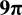# Diagonal of a Square

Back to “Geometry” Page

The diagonal of a square is as simple as the Pythagorean theorem.

It is very simple:

(length)2 + (width)2 = (diagonal)2

In other words, you simply take the square root of the sum of the squares of the length and width of the square.

(length)2 + (width)2   = d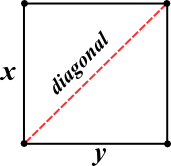A simple equation for the diagram above would be this:

x2 + y2   = d

Since the length and width of a square are equal, you can simply multiply one side squared by 2, like this:

2(x2) = d

So, if a side of the square above is equal to 1, then the equation for the diagonal is this:

2(12) = d

2  = d

Incidentally,  2  is approximately 1.4. That’s a handy number to remember.

And, if a side of the square above is equal to 2, then the equation for the diagonal is this:

2(22) = d

2(4)  = d

2 2  = d

And, if a side of the square above is equal to 3, then the equation for the diagonal is this:

2(32) = d

2(9)  = d

3 2  = d

You can now see a pattern developing. The diagonal of a square is equal to any side of the square times  2 .

See whether you can solve the flashcards below.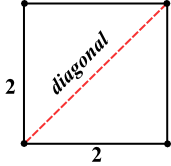• ﻿What is the diagonal of the square above?;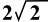• What is the area of the square above?; 4
• What is the perimeter of the square above?; 8
• What's the area of the isoceles triangle?; 2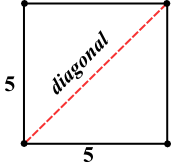• ﻿What is the diagonal of the square above?;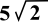• What is the area of the square above?; 25
• What is the perimeter of the square above?; 20
• What's the area of the isoceles triangle?; 12 1/2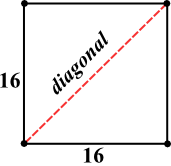• ﻿What is the diagonal of the square above?;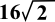• What is the area of the square above?; 256
• What is the perimeter of the square above?; 64
• What's the area of the isoceles triangle?; 128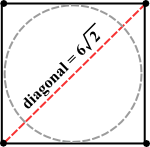Assume that the circle touches all four sides of the square in the diagram above.

• ﻿What is the diameter of the circle above?; 6
• What is the area of the square above?; 36
• What is the perimeter of the square above?; 24
• What's the circumference of the circle?;• What's the area of the circle?;